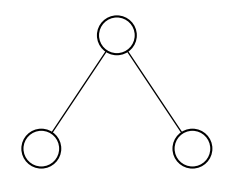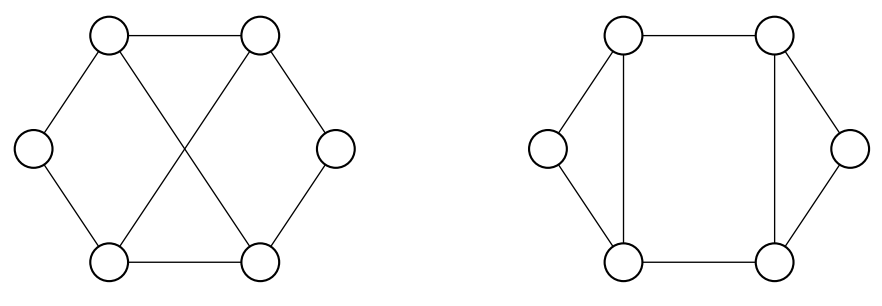시간 제한메모리 제한제출정답맞은 사람정답 비율
2 초 512 MB111100.000%

## 문제

Graph isomorphism is an important problem in Computer Science. It is not known whether the polynomial algorithm exists for this problem, neither it is known to be NP-complete.

Two undirected graphs $G$ and $H$ are called isomorphic if they have the same number of vertices and there exists a bijection $\varphi:VG \to VH$ such that there is an edge $uv$ in $G$ if and only if there is an edge $\varphi(u)\varphi(v)$ in $H$. There are some characteristics of graphs that are invariant under isomorphism. One of such parameters is degree profile of the graph.

The degree $\deg(u)$ of a vertex $u$ is the number of other vertices connected to $u$ by edges. Consider a connected undirected graph $G$ with $n$ vertices. For each vertex $u$ find sets $V_{u,0}, V_{u,1}, \ldots, V_{u, n-1}$ of vertices at distance $0, 1, \ldots, n-1$ from $u$ (some of these sets may be empty). For each such set find the multiset $D_{u, i}$ of degrees of vertices from $V_{u,i}$. The list of these multisets $D_u = [D_{u, 0}, D_{u, 1}, \ldots, D_{u, n-1}]$ is the degree profile of vertex $u$. The multiset of degree profiles of all vertices of the graph is its degree profile.

For example, the graph displayed below has degree profile $\big\{[\{1\},\{2\},\{1\}], [\{2\},\{1,1\},\varnothing], [\{1\},\{2\},\{1\}]\big\}$.It is clear that degree profile is invariant under isomorphism. However, there can be graphs that have the same degree profile but are not isomorphic. The example of two such graphs is shown on the picture below. Degree 2 vertices of both graphs have degree profiles $[\{2\}, \{3, 3\}, \{3, 3\}, \{2\}, \varnothing, \varnothing]$, and degree 3 vertices have degree profiles $[\{3\}, \{2, 3, 3\}, \{2, 3\}, \varnothing, \varnothing, \varnothing]$, but graphs are clearly not isomorphic.Note that when different degree profiles prove that graphs are not isomorphic, same degree profiles do not give easy way to find isomorphism even if it exists, because correspondence between vertices of the same degree profile can be difficult to establish. There is however class of graphs for which degree profile allows to easily check for isomorphism. These are graphs where all vertices have different degree profiles. Let us call such graphs degree distinguishable.

However, even degree distinguishable graphs can have the same degree profile but be non-isomorphic. Given $n$ find two non-isomorphic connected degree distinguishable graphs with $n$ vertices that have the same degree profile.

## 입력

The input file contains one integer $n$ ($3 \le n \le 100$).

## 출력

If there are no two non-isomorphic degree distinguishable graphs with $n$ vertices that have the same degree profile, print "NO" at the first line of the output file. In the other case print "YES" followed by two graph description.

Each description must start with $m$ --- number of edges, followed by $m$ pairs of integers: pairs of vertices connected by edges. There must be at most one edge between a pair of vertices, no edge must connect a vertex to itself.

Vertices of each graph must be numbered from 1 to $n$ in such way that vertices $i$ of both graphs have the same degree profile. No two vertices of the same graph must have the same degree profile.

## 예제 입력 1

3


## 예제 출력 1

NO


## 예제 입력 2

6


## 예제 출력 2

YES
8
1 2
1 6
2 3
2 5
3 4
3 6
4 5
5 6
8
1 2
1 6
2 3
2 6
3 4
3 5
4 5
5 6


Note that this is incorrect output.

## 노트

The second example gives two non-isomorphic graphs with the same degree profile but not degree distinguishable. They are provided to illustrate output format, but such output for $n = 6$ will not be accepted.

## 채점 및 기타 정보

• 예제는 채점하지 않는다.